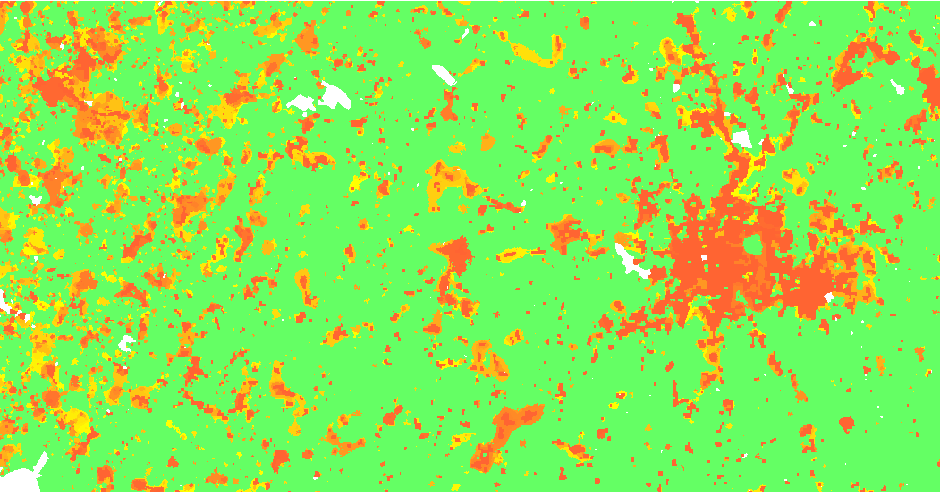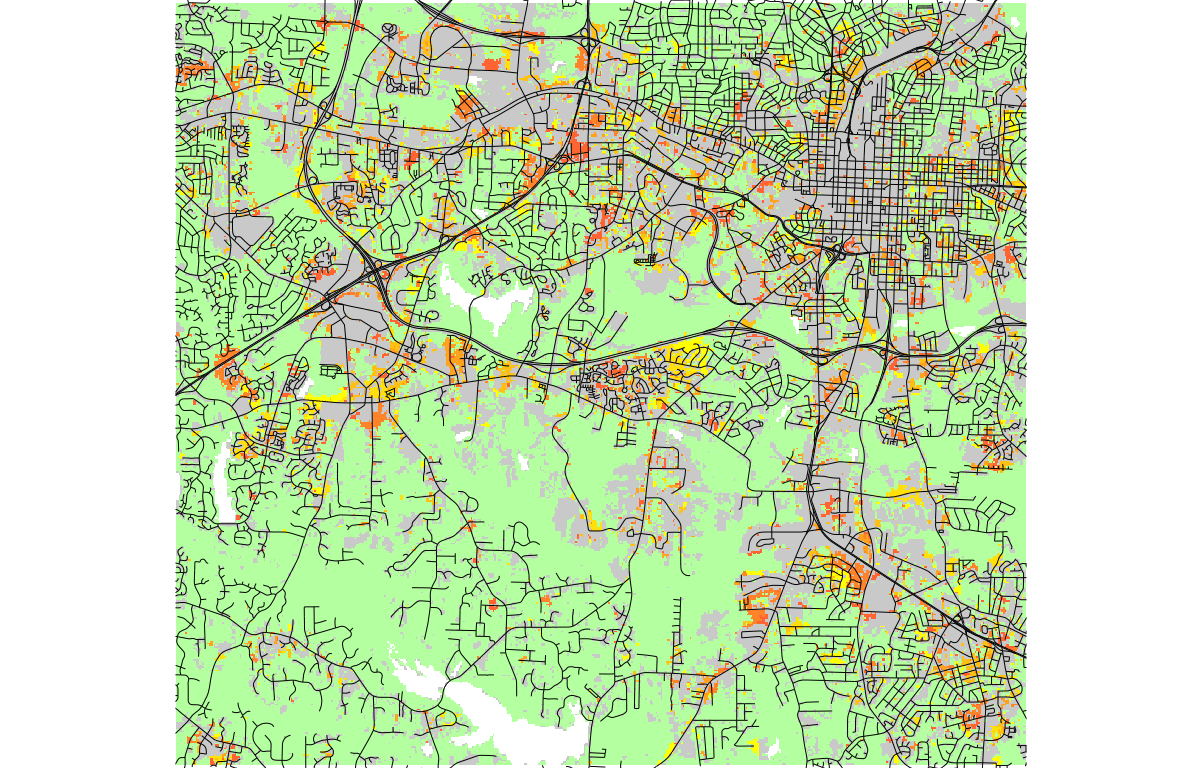## NAME

r.futures - GRASS GIS Reference Manual

## DESCRIPTION

r.futures.* is an implementation of FUTure Urban-Regional Environment Simulation (FUTURES) which is a model for multilevel simulations of emerging urban-rural landscape structure. FUTURES produces regional projections of landscape patterns using coupled submodels that integrate nonstationary drivers of land change: per capita demand (DEMAND submodel), site suitability (POTENTIAL submodel), and the spatial structure of conversion events (PGA submodel).

### Submodels

DEMAND
DEMAND estimates the rate of per capita land consumption specific to each subregion. Projections of land consumption are based on extrapolations between historical changes in population and land conversion based on scenarios of future population growth. How to construct the per capita demand relationship for subregions depends on user's preferences and data availability. Land area conversion over time can be derived for the USA, e.g. from National Land Cover Dataset. A possible implementation of the DEMAND submodel is available as module r.futures.demand.
POTENTIAL
The POTENTIAL submodel uses site suitability modeling approaches to quantify spatial gradients of land development potential. The model uses multilevel logistic regression to account for hierarchical characteristics of the land use system (variation among jurisdictional structures) and account for divergent relationships between predictor and response variables. To generate a binary, developed-undeveloped response variable using a stratified-random sample, see module r.sample.category. The coefficients for the statistical model that are used to calculate the value of development potential can be derived with module r.futures.potential, which uses multilevel logistic regression in R. One of the predictor variables is development pressure (computed using r.futures.devpressure) which is updated each step and thus creates positive feedback resulting in new development attracting even more development.
PGA
Patch-Growing Algorithm is a stochastic algorithm, which simulates undeveloped to developed land change by iterative site selection and a contextually aware region growing mechanism. Simulations of change at each time step feed development pressure back to the POTENTIAL submodel, influencing site suitability for the next step. PGA is implemented in r.futures.pga.Figure: FUTURES submodels and input data

### Input data

We need to collect the following data:
Study extent and resolution
Specified with g.region command.
Subregions
FUTURES is designed to capture variation across specified subregions within the full study extent. Subregions can be for example counties. DEMAND and POTENTIAL can both be specified according to subregions. Subregion raster map contains the subregion index for each cell as integer starting from 1. If you do not wish to model by subregion, all values in this map should be 1.
Population data
DEMAND submodel needs historical population data for each subregion for reference period and population projections for the simulated period.
Development change
Based on the change in developed cells in the beginning and end of the reference period, and the population data, DEMAND computes how many cells to convert for each region at each time step. Development change is also used for deriving the patch sizes and shape in calibration step (see r.futures.calib) to be used in PGA submodel. DEMAND and PGA require a raster map representing the starting state of the landscape at the beginning of the simulation (developed = 1, available for development = 0, excluded from development as NULLs).
Predictors
Development potential (POTENTIAL submodel) requires a set of uncorrelated predictors (raster maps) driving the land change. These can include distance to roads, distance to interchanges, slope, ...
Development pressure
The development pressure variable is one of the predictors, but it is recalculated at each time step to allow for positive feedback (new development attracts more development). For computing development pressure, see r.futures.devpressure.Figure: FUTURES simulation result

## EXAMPLE

Simple example using nc_spm_08_grass7 dataset. Please see tutorials on GRASS wiki for more realistic examples.

Create rasters representing urbanization using NDVI, exclude lakes:

```g.region raster=lsat7_2002_30@PERMANENT
i.vi red=lsat7_2002_30@PERMANENT output=ndvi_2002 nir=lsat7_2002_40@PERMANENT
i.vi red=lsat5_1987_30@landsat output=ndvi_1987 nir=lsat5_1987_40@landsat
r.mapcalc expression="urban_1987 = if(ndvi_1987 <= 0.1 && isnull(lakes), 1, if(isnull(lakes), 0, null()))"
r.mapcalc expression="urban_2002 = if(ndvi_2002 <= 0.1 && isnull(lakes), 1, if(isnull(lakes), 0, null()))"
```
Create predictors - slope, distance from lakes in km, distance from roads in km, development pressure:
```r.slope.aspect elevation=elevation slope=slope

r.grow.distance input=lakes distance=lakes_dist
r.mapcalc "lakes_dist_km = lakes_dist/1000."

v.to.rast input=streets_wake output=streets use=val
r.grow.distance input=streets distance=streets_dist
r.mapcalc "streets_dist_km = streets_dist/1000."

r.futures.devpressure input=urban_2002 output=devpressure method=gravity size=15 -n
```
Sample predictors and developed areas:
```r.sample.category input=urban_2002 output=sampling sampled=slope,lakes_dist_km,streets_dist_km,devpressure,zipcodes npoints=300,100
```
Compute POTENTIAL regression coefficients, using zipcodes as subregion:
```r.futures.potential input=sampling output=potential.csv columns=devpressure,slope,lakes_dist_km,streets_dist_km developed_column=urban_2002 subregions_column=zipcodes
```
Compute how many cells should be converted in each subregion:
```r.futures.demand development=urban_1987,urban_2002 subregions=zipcodes observed_population=observed_population.csv projected_population=projected_population.csv \
simulation_times=2003,2004,2005,2006,2007,2008,2009,2010 method=linear,logarithmic,exponential demand=demand.csv
```
Create a list of patch sizes (here we skip calibration of patch sizes for simplicity):
```r.futures.calib -l development_start=urban_1987 development_end=urban_2002 patch_threshold=0 patch_sizes=patches.txt subregions=zipcodes --o
```
Run patch growing to get final results:
```r.futures.pga developed=urban_2002 subregions=zipcodes output=futures output_series=futures predictors=slope,lakes_dist_km,streets_dist_km devpot_params=potential.csv \
development_pressure=devpressure n_dev_neighbourhood=15 development_pressure_approach=gravity gamma=1.5 scaling_factor=1 demand=demand.csv discount_factor=0.1 \
compactness_mean=0.4 compactness_range=0.05 num_neighbors=4 seed_search=probability patch_sizes=patches.txt random_seed=1
```Figure: One stochastic realization of FUTURES simulation, orange to yellow gradient represents new development where yellow is the latest.

## REFERENCES

r.futures.pga, r.futures.parallelpga, r.futures.devpressure, r.futures.calib, r.futures.demand, r.futures.potential, r.futures.potsurface, r.sample.category

## AUTHORS

Corresponding author:
Ross K. Meentemeyer, rkmeente ncsu edu, Center for Geospatial Analytics, NCSU

Original standalone version:
Ross K. Meentemeyer *
Wenwu Tang *
Monica A. Dorning *
John B. Vogler *
Nik J. Cunniffe *
Douglas A. Shoemaker *
Jennifer A. Koch **

* Department of Geography and Earth Sciences, UNC Charlotte
** Center for Geospatial Analytics, NCSU

Port to GRASS GIS and GRASS-specific additions:
Vaclav Petras, NCSU GeoForAll

Development pressure, demand and calibration and preprocessing modules:
Anna Petrasova, NCSU GeoForAll

## SOURCE CODE

Available at: r.futures source code (history)

Latest change: Wed Mar 30 10:17:35 2022 in commit: 25b0a9981b66c443a1c1af1d5f26182c93268b45

© 2003-2022 GRASS Development Team, GRASS GIS 8.0.3dev Reference Manual